# CSC301: Week 1: Trees, iteration and recursion (3.2)

 Contents [0/56]

 Are You In The Right Room? [1/56]

Course: CSC301 (Data Structures II)

Instructor: James Riely

 Overview of Today's Class [2/56]

• Homework!
• Binary search trees

This is the second course in a two-course sequence on data structures using Java. The course focuses mainly on the following data structures, their analysis, and their applications: trees (search trees, balanced search trees), heaps, associative arrays, hash tables, and data structures for representing graphs. The implementation of the basic operations on each data structure are discussed and analyzed in terms of their efficiency. The applications discussed highlight and exploit the unique characteristics of the different data structures, and emphasize problem solving and recursive thinking.

From Wired Magazine: Code is far from a utilitarian means to an end. Like painting or sculpting, it's a medium with which you can create something. And as such, code can take many forms: beautiful or ugly, elegant or clunky.

• Basic OO programming techniques
• Use of debuggers
• Use of testing
• Understanding correctness of programming
• Understanding performance characteristics of programs
• Competence with iterative and recursive solutions to problems

Plus

• Understanding of trees.
• Understanding of hash tables.
• Understanding of graphs.
• Understanding of string algorithms.

CSC300/402 C- or better.

You must attend class!

• Retro-Active Withdrawal

CDM understands certain extenuating circumstances can hinder one's ability for academic success and completion of course work. Please see http://www.cdm.depaul.edu/Current%20Students/Pages/Enrollment-Policies.aspx for additional information.

In order to petition for an excused absence, students who miss class due to illness or significant personal circumstances should complete the Absence Notification process through the Dean of Students office. The form can be accessed at http://studentaffairs.depaul.edu/dos/forms.html. Students must submit supporting documentation alongside the form. The professor reserves the sole right whether to offer an excused absence and/or academic accommodations for an excused absence.

You must subscribe to the course discussion forum on google groups. Do it as soon as possible.

• Don't troll.
• Please don't send thank you messages to the group.

The discussion forum is an extension our time in class. This is particularly great for students that miss the live lecture. If you are watching the class online, you should write down any questions that arise, including the time from the recording for reference. Then send the list of questions to me, and I will post a reply to the group.

I will also use Teamviewer for communication. With teamviewer, you can show me your computer screen while we talk over the phone.

Grades will be determined as follows.

• 3% Participation (In-class students)
• 20% Online quizzes
• 25% Programming assignments
• 52% Exams

You must pass the final exam in order to pass the course.

For in class students: Participation will consist of me calling on students in class. If you are not here or don't hear, then you will lose participation credit.

Course homepage: http://fpl.cs.depaul.edu/jriely/ds2/

• Lecture slides may not be available before the class.
• Lecture slides may change after class.

 Required Books
 by Cay Horstmann (Addison-Wesley, 2017) Available as Ebook Available online via Safari Older edition is fine.
 by Robert Sedgewick and Kevin Wayne (Addison-Wesley, 2011) Available as Ebook Available online via Safari Do not get an older edition. They are completely different books.
 Recommended Books
 by John Hubbard (Schuams, 2009) This book is a good source of example problems with solutions. Available as Ebook
 More books
 by Robert Sedgewick and Kevin Wayne (Addison-Wesley, 2011) Available as Ebook
 How to Think Like a Computer Scientist by Allen B. Downey. Free! An good introduction to Java. Skip the GridWorld chapters, which are intended to help with the AP exam in CS. See also these lecture notes from MIT. The first three lectures are particularly useful.
 Introduction to Programming in Java (Chapter 1) by Robert Sedgewick and Kevin Wayne Free! This is the first chapter of the introductory text written by the authors of our primary textbook. It presents the same material as section 1.1 of the primary text, but at a slower pace.
 by Joshua Bloch (Addison-Wesley, 2008) Available as Ebook Available online via Safari

The algorithms text describes all of the Java that is required for the class. The discussion is terse, making it an excellent reference. If you would like a longer discussion of Java, you might want a supplementary text. In this case, you might consider one of the following.

• Kathy Sierra and Bert Bates's "Head First Java" (on Safari)
• David Flanagan's "Java in a Nutshell" (on Safari)
• Cay Horstman's "Core Java I" (on Safari)
• Cay Horstman's "Big Java" (on Safari)

We will discuss concepts in class.

You will have weekly programming assignments.

• You do not have to do it alone, but you must be self-motivated.
• You can ask me and other members of the class.
• You must start assignments early.

Getting the homework correct is not enough. The first time you solve something it could take days, and may require help. Once you get a solution, you are not done.

• First, try to make your code as simple as possible. It should be self-explantory to an average programmer, and include no unnecessary conditionals or extra lines of code.
• Then, create a copy of the homework and erase your solutions.
• Upon waking the next day, attempt to redo the problems. Do not look at your previous solution. Do not ask for help, either from friends or from the internet.
• Repeat this process until you can write each single function correctly in five minutes or less. Only then have you internalized the problem.
• Try to invent a variant of the problem that is easy to solve once you know how to solve this one.

From the Chronicle of Higher Education: People often mistake familiarity for understanding. They open the textbook after getting home from a lecture, and they recognize the material. They think: I get this. Then they take a test -- and bomb it.

I do not give out solutions to homework problems.

• I will show every solution during lecture.
• What I suggest is that while watching you lecture, you pause on any code you'd like the solution for, and type it in.
• I firmly believe that you will learn something in the process, that you will not learn by simply looking at my solution.

How to succeed:

• Be present.
• Forgive yourself.

This step is only necessary to draw the pictures that I show in class.On the mac, you need to install Homebrew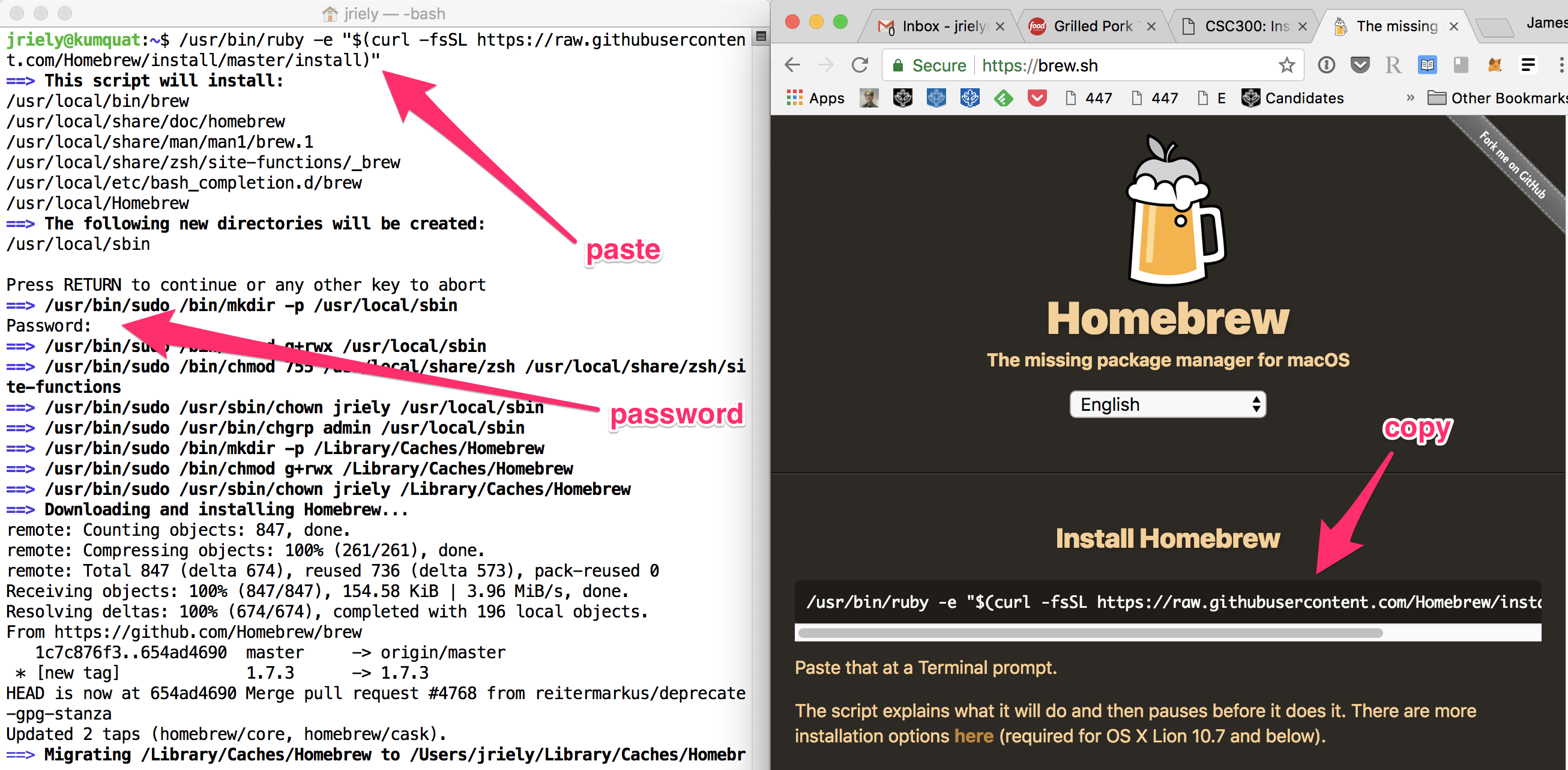Once you have homebrew, just enter the following commands on the Terminal:

```brew update
brew install graphviz
```

 Admin: Install code for this class [13/56]

Step-by-step:
• Create a folder structure for course materials

For example, you might use:

```  C:\Users\myname\Documents\DataStructures
```

Unzip the file into the folder for class.

This should create a folder called `eclipse-workspace`.

The `eclipse-workspace` folder should contain `algs4`.

The `algs4` folder should contain `data`, `lib`, `src`, and maybe some other things.

Verify that the folder hierarchy looks like this:

```  C:\Users\myname\Documents\DataStructures\eclipse-workspace
C:\Users\myname\Documents\DataStructures\eclipse-workspace\algs4
C:\Users\myname\Documents\DataStructures\eclipse-workspace\algs4\data
C:\Users\myname\Documents\DataStructures\eclipse-workspace\algs4\lib
C:\Users\myname\Documents\DataStructures\eclipse-workspace\algs4\src
```

On my machine, I have the following:We will use Java 11. It is easiest to use eclipse if you remove any other versions of Java from your machine.

• For windows, use the uninstaller to remove other Java versions.
• For mac, use the Finder to remove other Java versions in `/Library/Java/JavaVirtualMachines/`

JDK stands for Java Development Kit.

You should get Eclipse IDE for Java Developers. The main difference between versions is the number of packages that come pre-installed. This is the smallest version that has everything we need.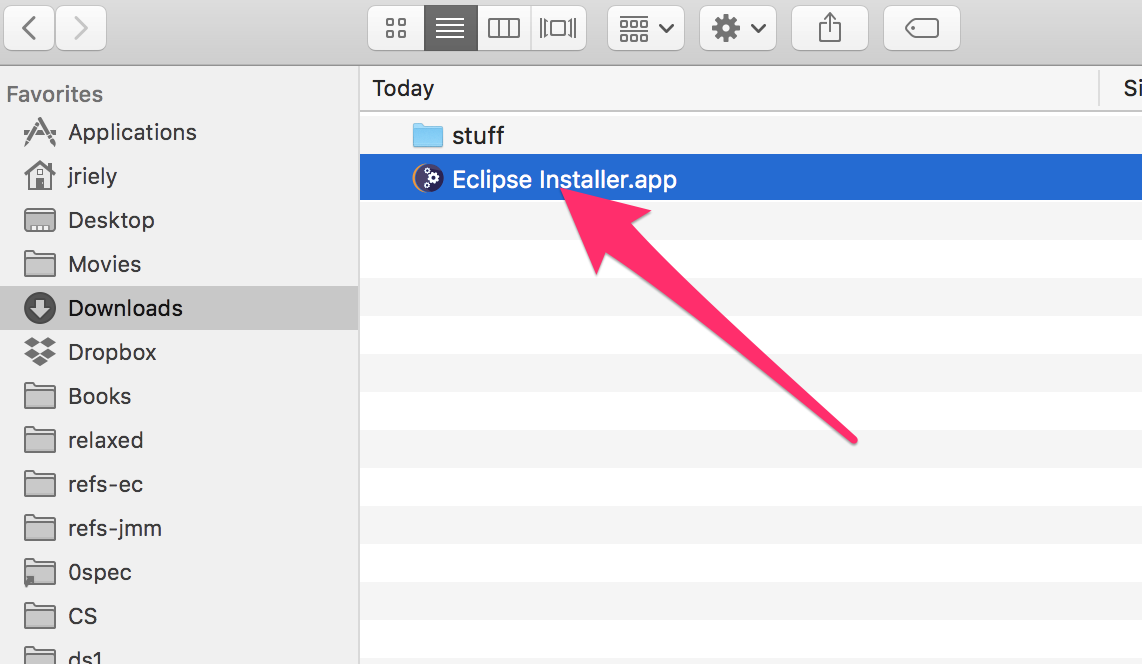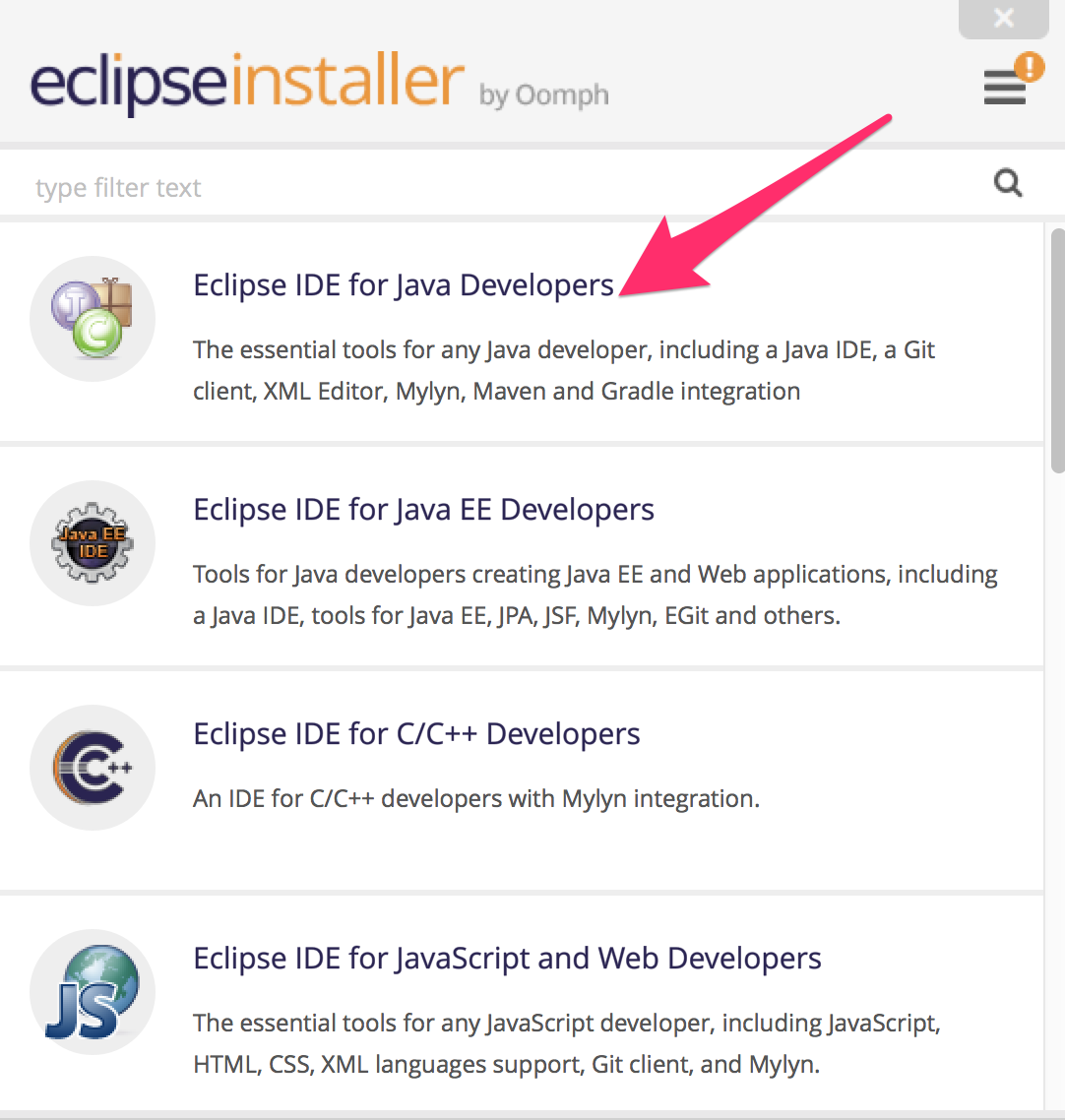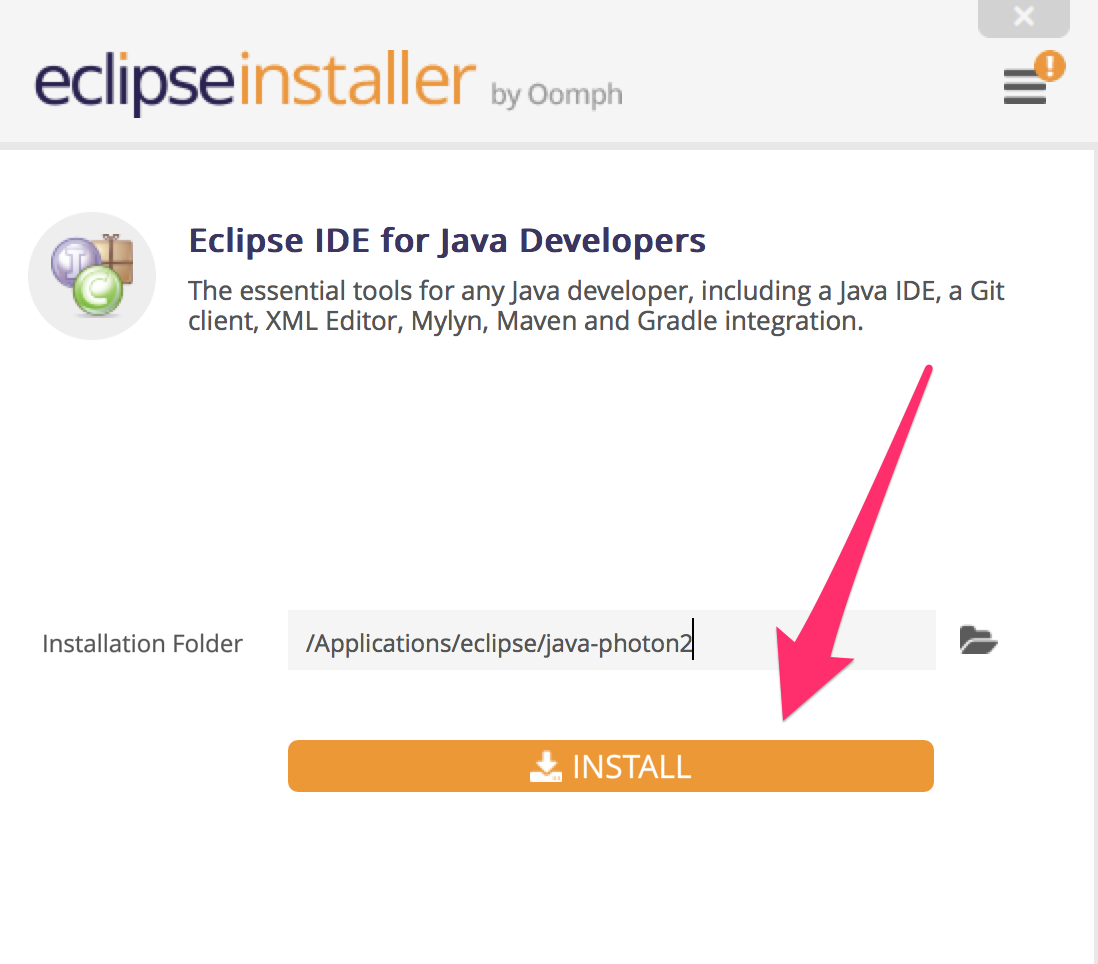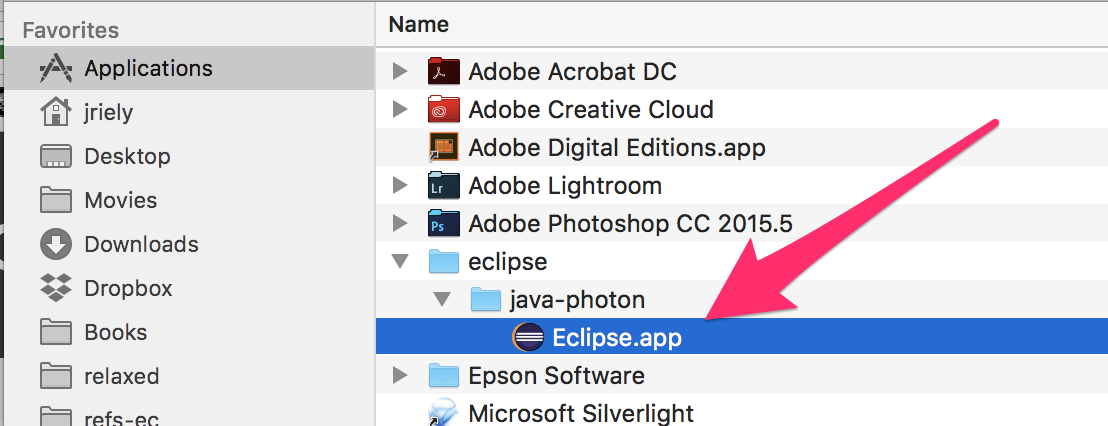There is a video walking through these steps on windows: Algs4 workspace movie

• Make sure that you have downloaded the code for the class, as instructed before.

Verify that the folder hierarchy looks like this:

```  C:\Users\myname\Documents\DataStructures\eclipse-workspace
C:\Users\myname\Documents\DataStructures\eclipse-workspace\algs4
C:\Users\myname\Documents\DataStructures\eclipse-workspace\algs4\data
C:\Users\myname\Documents\DataStructures\eclipse-workspace\algs4\lib
C:\Users\myname\Documents\DataStructures\eclipse-workspace\algs4\src
```

On my machine, I have the following:• Start eclipse. When it prompts you for a eclipse-workspace, select your `eclipse-workspace` folder.

```  C:\Users\myname\Documents\DataStructures\eclipse-workspace
```

DO NOT SELECT THE `algs4` FOLDER!• In eclipse, navigate to

```  File > New > Java Project
```

In project name type `algs4`. and then click the finish buttonDisable warnings for unnecessary code. LEAVE ALL OTHER WARNINGS AT THEIR DEFAULT VALUES.On windows, the `"Preferences"` option is under `"Window"` instead of `"Eclipse"`

```  Go to "Eclipse/Window" > "Preferences" > "Java" > "Compiler" > "Errors/Warnings" > "Unnecessary code"
Set the following to "Ignore"
"Value of local variable is not used"
"Unused private member"
```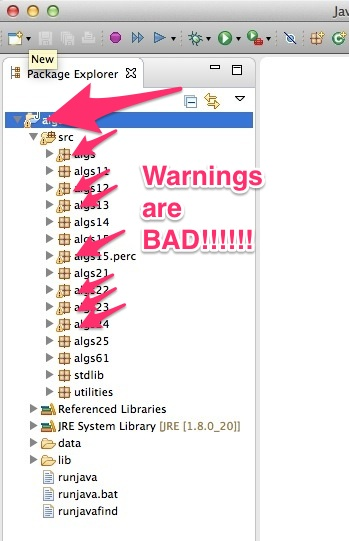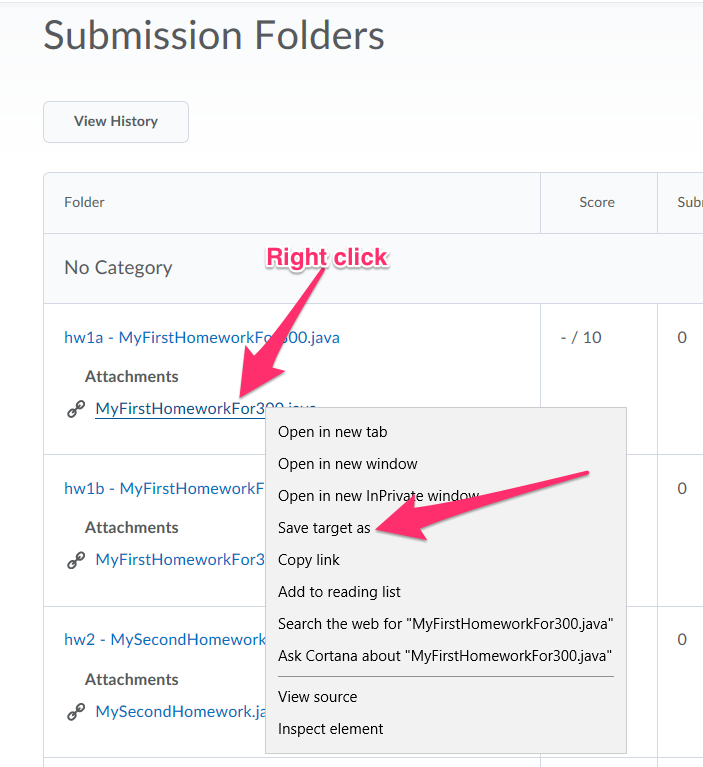Use drag-and-drop to add the file to eclipse into the correct package.If drag and drop are not working, try the following:

• Use the finder to drop the file into the eclipse-workspace. You need to put it in `eclipse-workspace\algs4\src\algs11`. Make sure there are `.java` files there, not `.class` files.
• After you have it there, in eclipse, go to the `File` menu and select `Refresh`.

After you have completed the assignment, save your work in eclipse and use drag-and-drop to upload the file to D2L.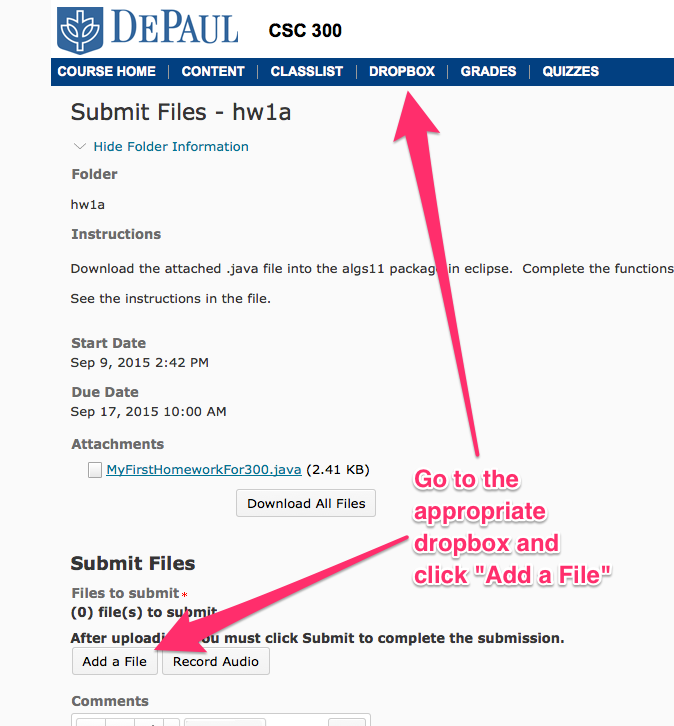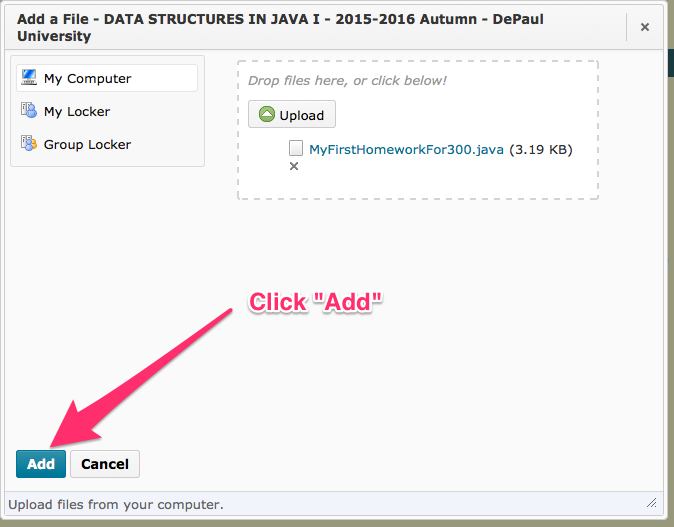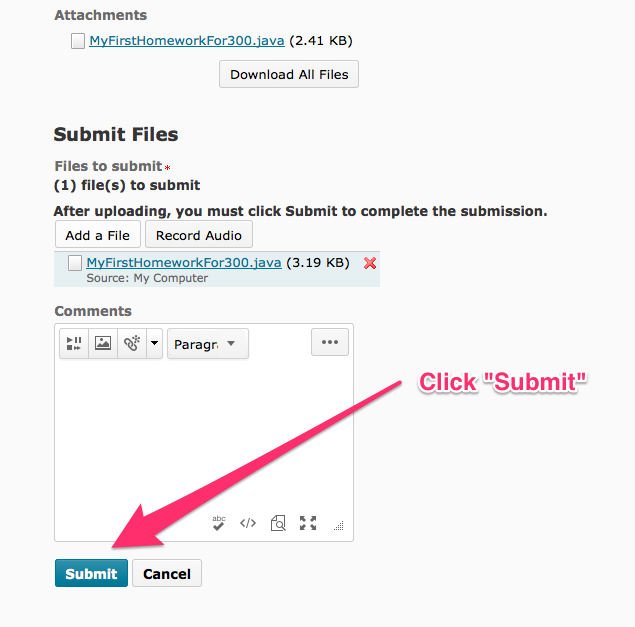If drag-and-drop does not work, you can use an alternative method, shown below.

Be very careful to upload a `.java` file from the `src` directory.

Do not upload a `.class` file from the `bin` directory.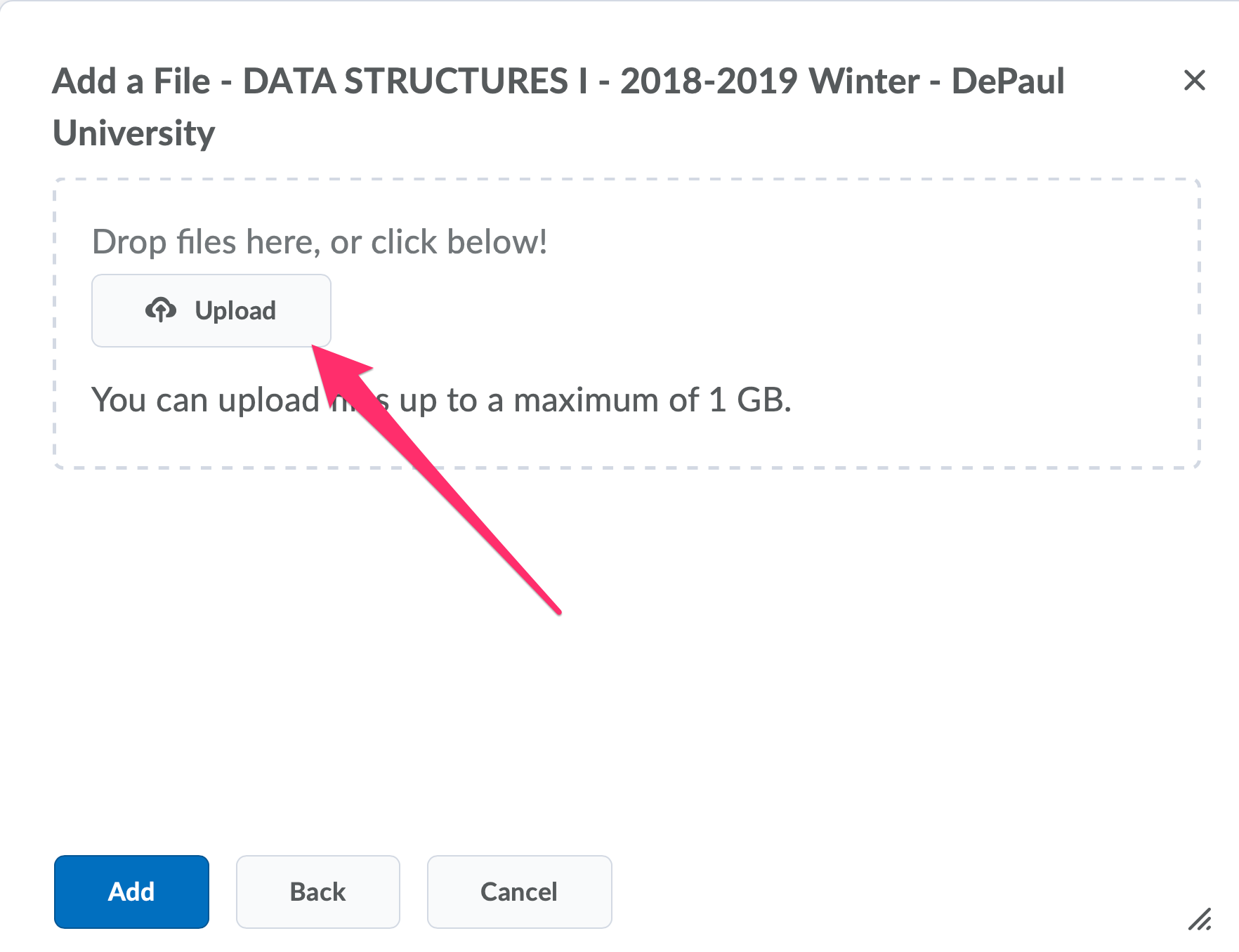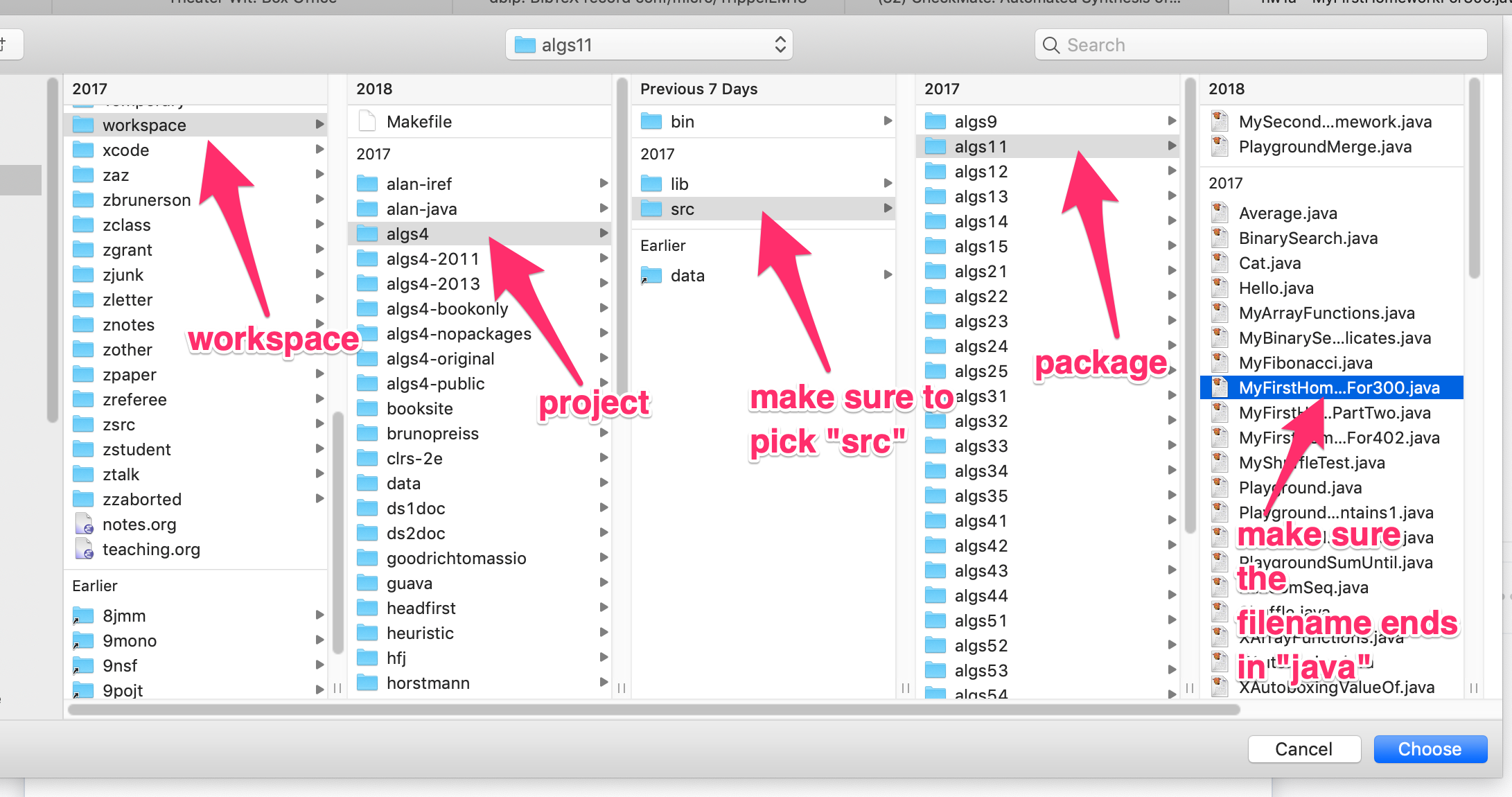If you have errors after installing eclipse and the code from class, please watch this: Eclipse problems

Uninstall everything and try again.

If that does not work, email me ASAP. Don't waste time trying to fix a broken eclipse install.

• describe the problem. More detail than eclipse is messed up.
• including a screenshot of eclipse showing the problem

 Admin: What to do if you get errors on the format function [21/56]

You may get a compiler error message like this:

```The method format(String, Object[]) in the type StdOut is not applicable for the arguments (String, String)
The method format(String, Object[]) in the type StdOut is not applicable for the arguments (String, String, int, int)
```

Make sure that you are using Java 11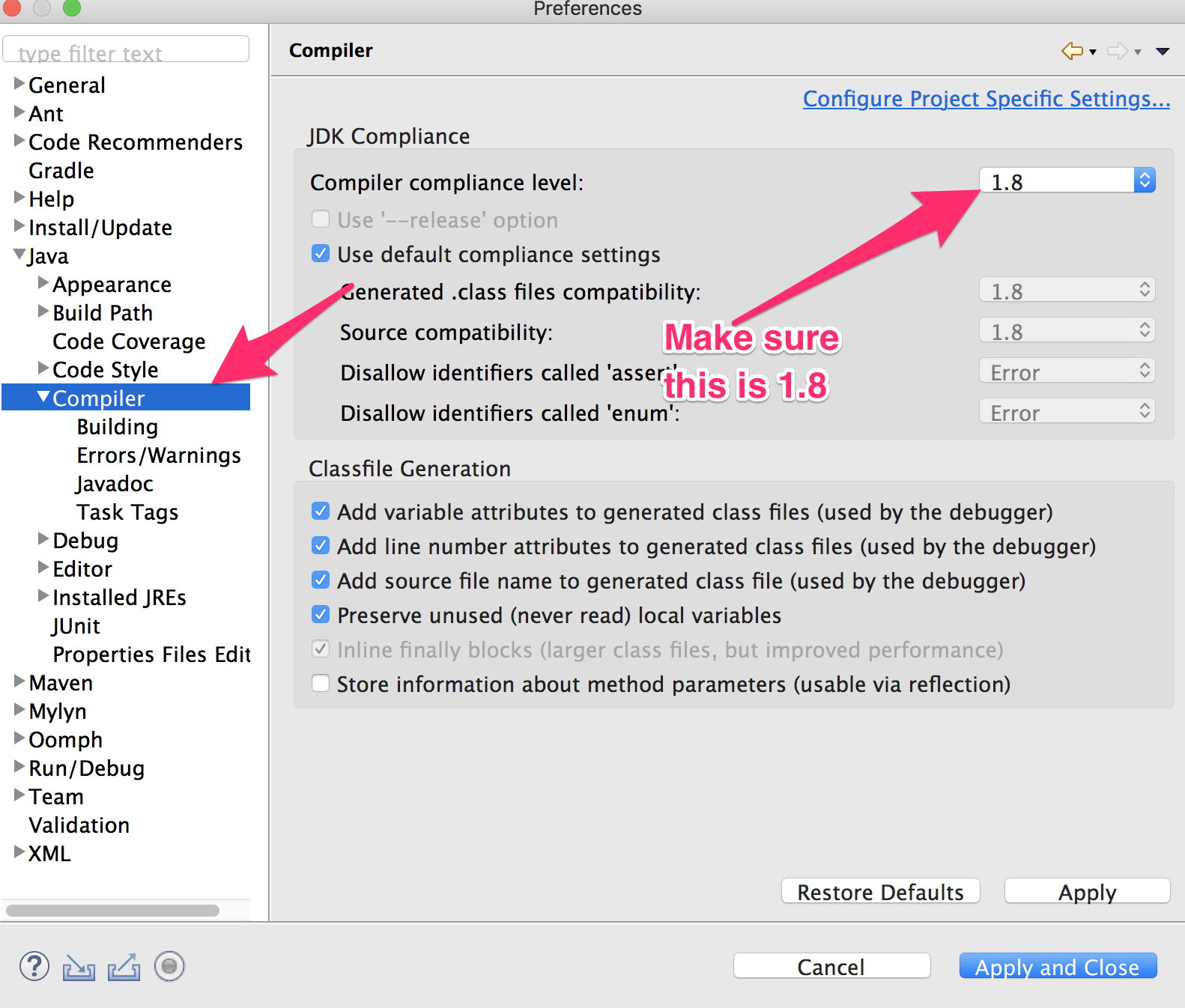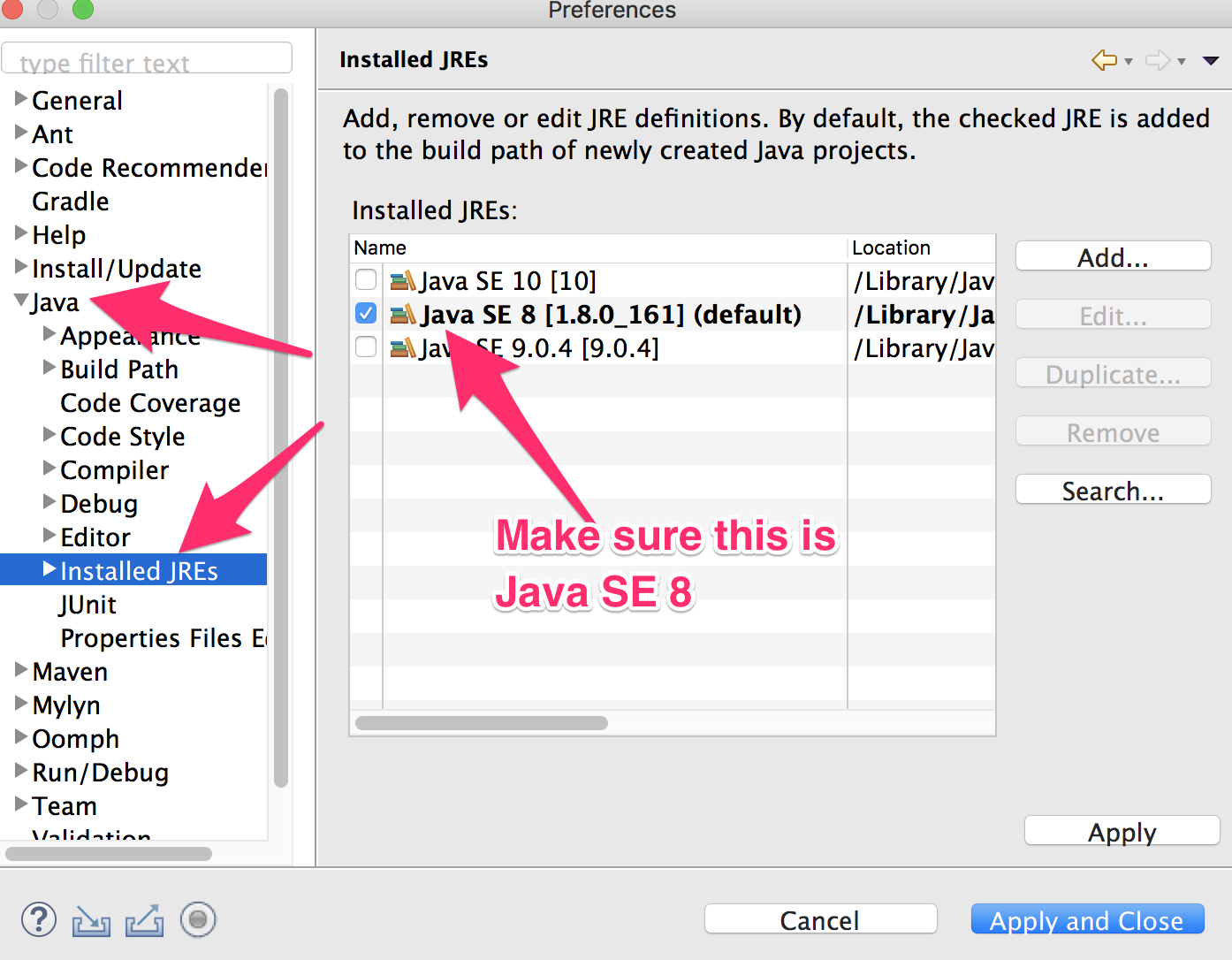Admin: What to do you have lots of red marks in Eclipse [22/56]

Suppose you had eclipse working and then one day, eclipse looks like this: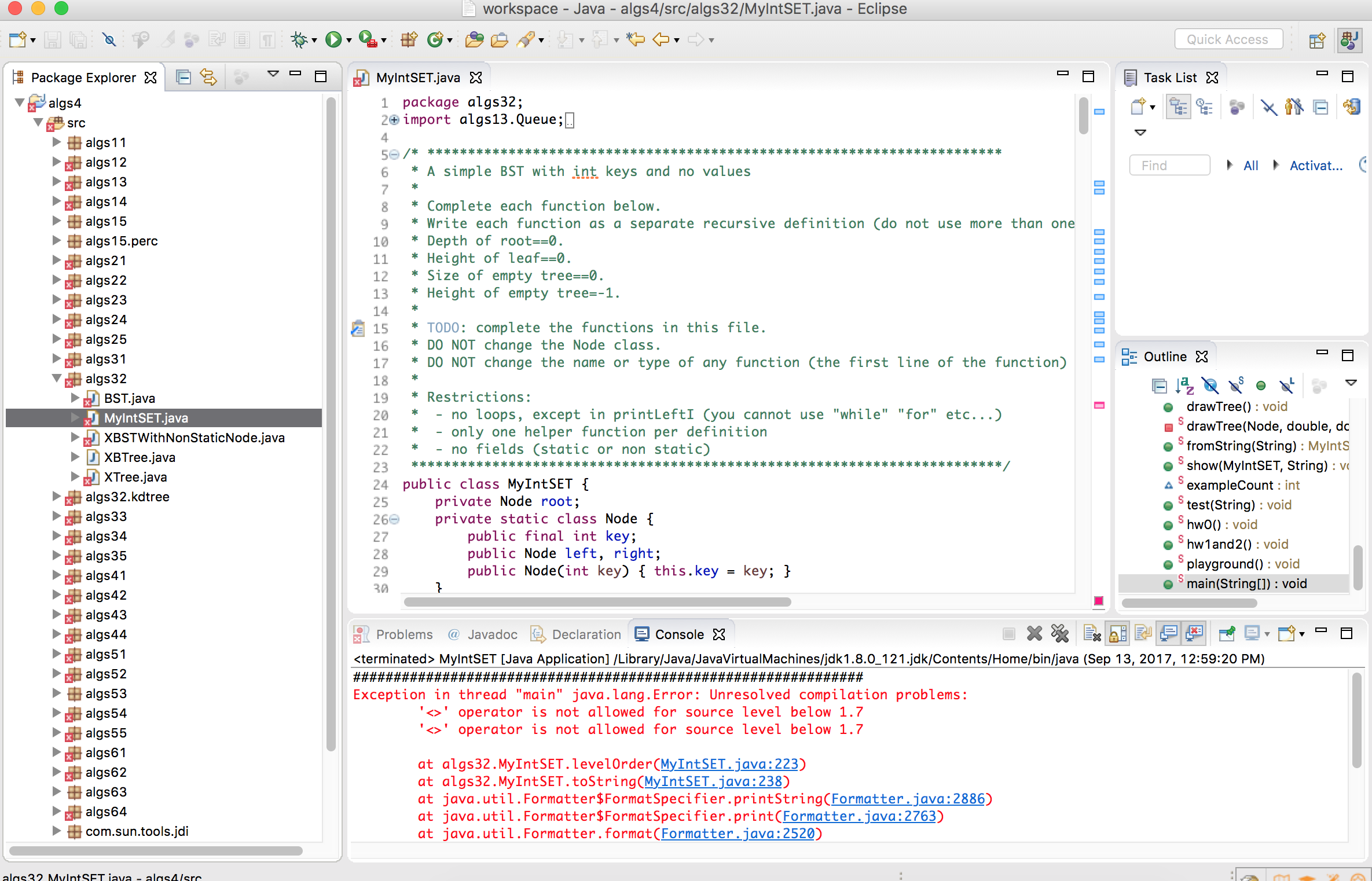Try

`  "Project" > "Clean..." > "Clean all projects"`

If that does not work, try creating a fresh eclipse-workspace.

• Exit eclipse.
• Using the finder, go to the folder that contains your `eclipse-workspace`.
• Rename it to `oldworkspace` and create a new folder called `eclipse-workspace`
• Inside the new `eclipse-workspace`, create a new folder called `algs4`
• Move the following folders from `oldworkspace/algs4` to `eclipse-workspace/algs4`
```data
lib
src
```
• Now start eclipse in the new "eclipse-workspace". It should be as though you are starting eclipse for the first time. Follow the instructions provided earlier to add the "algs4" project and to turn off the warnings for unused variables and private fields.

The above works by copying the good files to a new eclipse-workspace. You can also try the following approach, which removes the problemantic files from the exiting eclipse-workspace instead.

• Exit eclipse

• Using the finder or terminal, remove the following files/directories:

• `eclipse-workspace/.metadata`
• `eclipse-workspace/algs4/.classpath`
• `eclipse-workspace/algs4/.project`

If you are using finder on a mac, you may need to make these files visible. Run Terminal and enter the following two commands:

```defaults write com.apple.finder AppleShowAllFiles
TRUE killall Finder
```

To switch back, do the same but substitute `FALSE` for `TRUE`.

• Restart eclipse. You should have a blank eclipse-workspace.

• Follow the previous instructions to show the `algs4` project, by following the previous instructions to install the code from class, starting with ```File > New > Java Project```

If that does not work ensure that you have the correct version of Java installed.

• First check that Project Specific Settings are NOT checked in the following places
```Project -> Properties -> Java build path
Project -> Properties -> Java compiler```
• Then check
```Preferences -> Java -> Compiler
Preferences -> Java -> Installed JREs```
• to make sure that compliance level is set to 11 and that a Java SE 11 runtime is checked. If you don't have Java 11, then you need to install it.
• The clean all projects as described above.

Eclipse will only include JREs that were present before it started in a given eclipse-workspace. If you add a JRE later, the easiest thing to do is to recreate the eclipse-workspace from scratch. See the instructions on the previous slide.

You can also add a JRE after the fact, following these instructions: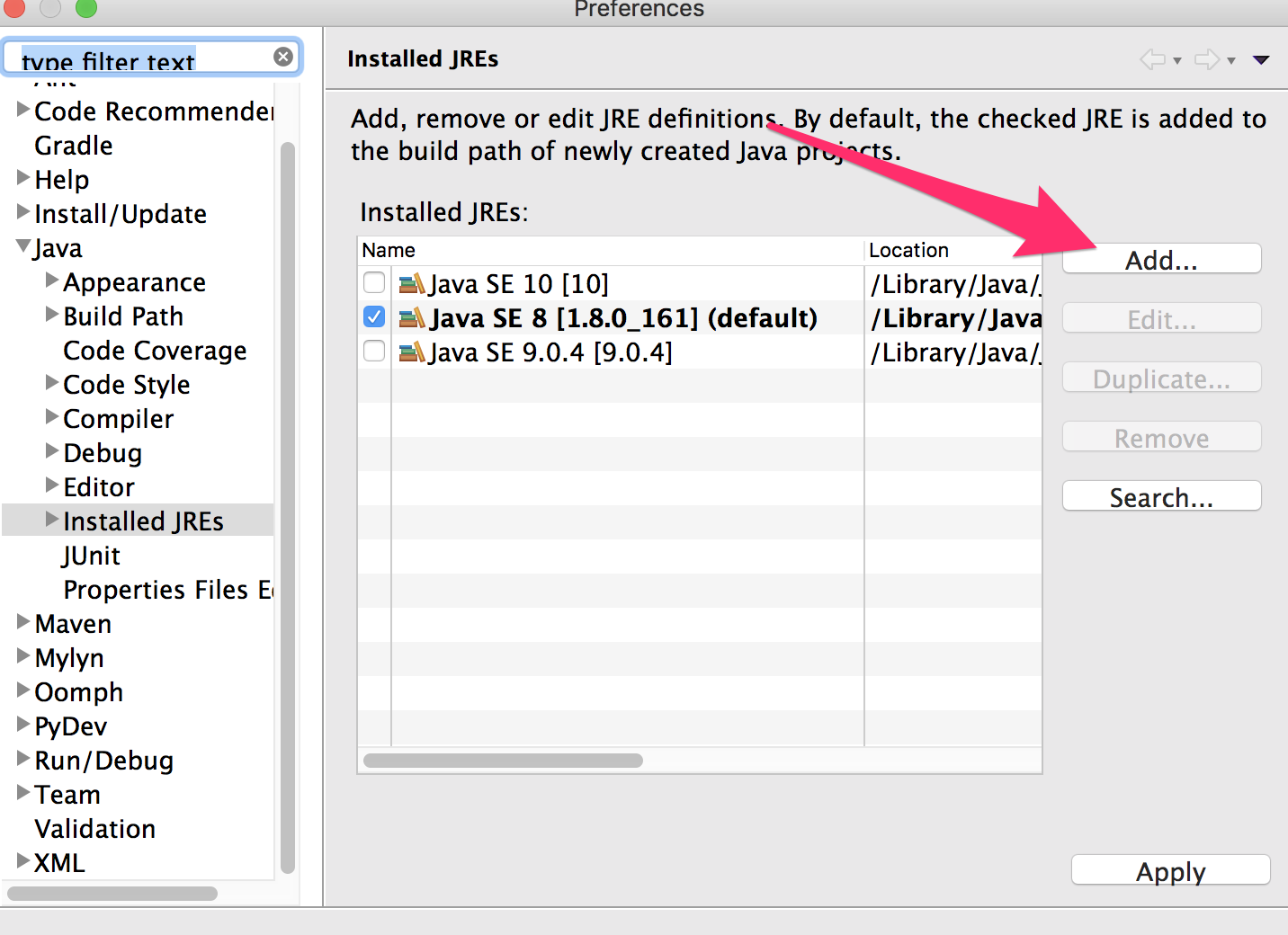Admin: What to do if you cannot see package explorer in Eclipse [24/56]

To show the package explorer, do this: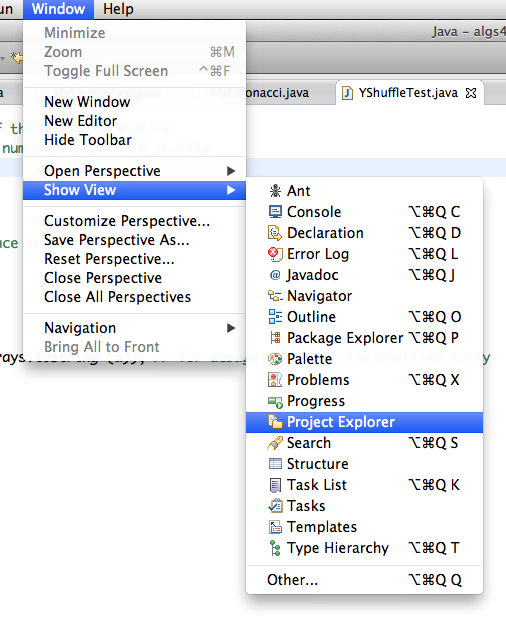Once it is showing, you can adjust the view using the buttons:You may get this Run As box in eclipse, like this: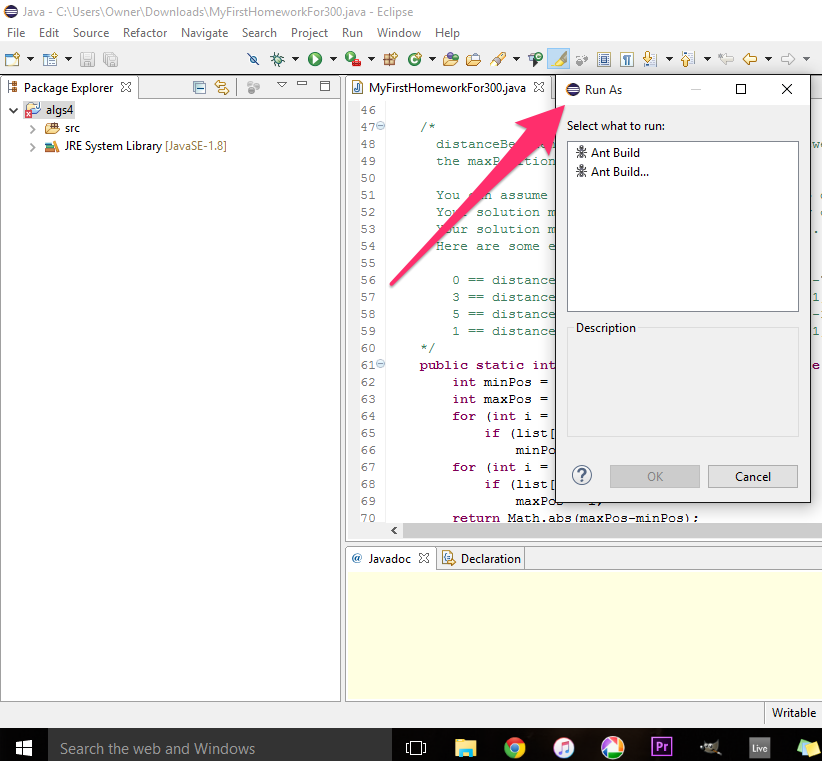Or this:First, make sure that you are running the code from the correct package in the src folder. Go back to the installation instructions for the code from class and make sure that the package explorer looks correct. If not, re-do the installation of the code from class, and start over.

If the package explorer looks okay, then try selecting the program you want to run in the package explorer and using a right click to bring up a context menu. You can select run from there:The run button is context sensitive in eclipse. It's behavior varies depending upon where the mouse was last clicked and what the last command to be run was. This problem usually sorts itself out. So try the run button again later.

If you want advice about an error, send me email, doing one of the following.

• Include a screenshot showing the error.

• Copy and paste the EXACT TEXT of the error into your email message.

• Include the code that caused the error, with enough context to understand it
• Make sure that you clearly indicate the line with the error.

If you have a problem getting a program to work and you want me to look at some of your code in more detail, send me email with the following three things.

1. Your java file as an attachment.

2. A description of how the output of the program is different from what you expected.

3. The output of your program, if it runs.

• Include in the screenshot, or
• Copy and poste from the eclipse console

The textbook code is written to run from the command line. We will not do this. Instead, we will edit the code in eclipse to provide the following, when necessary:

1. command line arguments
2. standard input
3. standard output

If the text runs the program:

```  java Program arg1 arg2 < input.txt > /tmp/output.txt
```

We will add the following lines to the "main" method of our java program:

```  public static void main(String[] args) {
args = new String[] { "arg1", "agrg2" };
StdIn.fromFile ("data/input.txt")
StdOut.toFile ("/tmp/output.txt")
...
```

 Homework [28/56]

• Look on D2L for quiz to complete.

• Look on D2L for homework to hand in.

• For Monday (due by 2:00pm):

Download `algs32.MyIntSET` from the dropbox on d2l. Add the function `printLeftI` that we discussed today in class and reupload.

You may add functions, but you must not remove or edit the name or signature of any function given in the file. Your code MUST COMPILE WITHOUT ERRORS. Code with compilation errors earns 0 points.

To put the skeleton files in eclipse, do the following.

• Download the `.java` attachments from d2l.

• Go to d2l.
• Click Dropbox in the menubar towards the top of the page, underneath the course title.
• Using the finder, drag the `.java` into the `algs32` package inside your eclipse workspace.

After you have completed the assignment, upload your completed `.java` file to the d2l dropbox.

• Go to d2l.
• Click Dropbox in the menubar towards the top of the page, underneath the course title.
• Drag the `.java` file from the eclipse package explorer onto the space on d2l.
• Submit the file.

Do not submit anything else. I will give zero credit for `.class` files. I will also give zero credit for any `.txt/.zip.rar`. Hand in a uncompressed `.java` source code file.

There will be no credit for code that has compilation errors (red marks in eclipse).

• For next wednesday (by 2:00pm):

Complete the `size` function in `algs32.MyIntSET` and reupload.

You may add functions, but you must not remove or edit the name or signature of any function given in the file. Your code MUST COMPILE WITHOUT ERRORS. Code with compilation errors earns 0 points.

• Skim Algorithms 3.1, 3.5

Check out the booksite. Read the summary of section 3.1 online before you read the textbook.

Check out the authors' coursesite for COS226 at Princeton. Find their lecture notes, homework assignments and old exams.

(The authors of the textbook also run classes on Coursera)

 Review Homework [29/56]

 Trees: Terminology [30/56]

• A root is a node with no ancestors.

• A leaf is a node with no descendants.

Note that in a singleton tree (tree with one node), the root is also a leaf.

• The size of a node is the number descendants (including itself).

A leaf node will have a size of 1.

The size of a tree is the size of its root. Empty tree has size of 0.

• The height of a node is the number of edges on the longest path from the node to a leaf.

A leaf node will have a height of 0.

The height of tree is the height of its root. Empty tree has height of -1.

• The depth of a node is the number of edges from the tree's root to the node.

A root node will have a depth of 0.

The depth of a tree is the same as it's height.Trees: Traversals [31/56]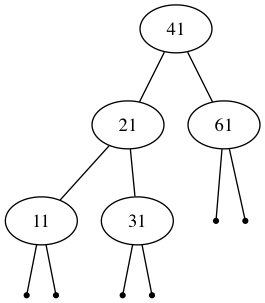• Level-order: 41 21 61 11 31

• In-order: (11 21 31) 41 61

• Pre-order: 41 (21 11 31) 61

• Post-order: (11 31 21) 61 41

Note: Parentheses are included only for clarity.

 Tree code: While loop going left [32/56]

```    public int sizeLeft () {
Node x = root;
int sz = 0;
while (x != null) {
x = x.left;
sz = sz + 1;
}
return sz;
}```

While loop going left.

Back to the size problem. Here is starter code which computes the size of the leftmost branch in the tree.

 Tree code: Recursion going left [33/56]

```    public int sizeLeft () {
return sizeLeft (root, 0);
}
private static int sizeLeft (Node x, int sz) {
if (x != null) {
sz = sizeLeft (x.left, sz + 1);
}
return sz;
}```

Recursion going left

 Tree code: Recursion going left and right [34/56]

```    public int size () {
return size (root, 0);
}
private static int size (Node x, int sz) {
if (x != null) {
sz = size (x.left, sz + 1);
sz = size (x.right, sz + 1);
}
return sz;
}```

Recursion going left and right. Now computing the size of the tree.

Is this correct?

 Tree code: Recursion going left and right [35/56]

```    public int size () {
return size (root, 0);
}
private static int size (Node x, int sz) {
if (x != null) {
sz = sz + 1;
sz = size (x.left, sz);
sz = size (x.right, sz);
}
return sz;
}```

What is the initial value of `sz` at each node as we go around the tree?

 Tree code: Recursion going left and right [36/56]

```    public int size () {
return size (root, 0);
}
private static int size (Node x, int sz) {
if (x != null) {
sz = sz + 1;
sz = size (x.left, sz);
sz = size (x.right, sz);
}
return sz;
}```

Computation of the size happens as we go forward, in a preorder traversal.

Initial value of `sz` at each node is the number of nodes that precede this one in a preorder traversal.

The initial value of `sz` does not include the node `x`.

Final value of `sz` is the initial value plus the size of the tree rooted at `x`: `size (x, sz)` returns size (`x`) + `sz`

 Tree code: Base case first (negating the conditional) [37/56]

```    public int size () {
return size (root, 0);
}
private static int size (Node x, int sz) {
if (x == null) return sz;
sz = sz + 1;
sz = size (x.left, sz);
sz = size (x.right, sz);
return sz;
}```

Base case first (negating the conditional)

This is more idiomatic for recursive functions.

 Tree code: Is this correct? [38/56]

```    public int size () {
return size (root, 0);
}
private static int size (Node x, int sz) {
if (x == null) return sz;
sz += 1;
sz += size (x.left, sz);
sz += size (x.right, sz);
return sz;
}```

Is this correct?

 Tree code: Is this correct? [39/56]

```    public int size () {
return size (root, 0);
}
private static int size (Node x, int sz) {
if (x == null) return sz;
sz += 1;
sz += size (x.left, sz);
sz += size (x.right, sz);
return sz;
}```

Is this correct?

No. `size (x, sz)` returns size (`x`) + `sz`, so this is adding it twice.

 Tree code: Is this correct? [40/56]

```    public int size () {
return size (root, 0);
}
private static int size (Node x, int sz) {
if (x == null) return sz;
sz = sz + 1;
size (x.left, sz);
size (x.right, sz);
return sz;
}```

Is this correct?

 Tree code: Is this correct? [41/56]

```    public int size () {
return size (root, 0);
}
private static int size (Node x, int sz) {
if (x == null) return sz;
sz = sz + 1;
size (x.left, sz);
size (x.right, sz);
return sz;
}```

Is this correct?

No. Mistake here is to think of `sz` as shared among the function calls.

 Tree code: Back to sanity! [42/56]

```    public int size () {
return size (root, 0);
}
private static int size (Node x, int sz) {
if (x == null) return sz;
sz = size (x.left, sz);
sz = size (x.right, sz);
sz += 1;
return sz;
}```

What are the initial values of `sz` as you go around the tree?

 Tree code: Make right call independent of the left [43/56]

```    public int size () {
return size (root, 0);
}
private static int size (Node x, int sz) {
if (x == null) return sz;
sz = size (x.left, 0);
sz += size (x.right, 0);
sz += 1;
return sz;
}```

Make it so that the right does not know about the left!

Change so that size returns just the size of `x`, rather than size of `x` plus `sz`

Intial value of `sz` is always 0

 Tree code: Don't need the sz parameter! [44/56]

```    public int size () {
return size (root);
}
private static int size (Node x) {
if (x == null) return 0;
int sz = 0;
sz += 1;
sz += size (x.left);
sz += size (x.right);
return sz;
}```

Don't need the sz parameter!

Node `x` is counted postorder, as we return.

This is true regardless of where we put `sz += 1`, since the intermediate values are not passed around.

 Tree code: Local variables don't matter [45/56]

```    public int size () {
return size (root);
}
private static int size (Node x) {
if (x == null) return 0;
int szl = size (x.left);
int szr = size (x.right);
return szl + szr + 1;
}```

Local variables don't matter.

This is static single assignment (SSA) form: each variable is assigned on exactly one line of code.

Most compilers translate your code into SSA as part of the compilation process.

In SSA, the right-hand-side of the assignment may be restricted to be either a single function call or a single operator. In this case, the last line would be translated to:

```        int tmp1 = szl + szr;
int tmp2 = tmp1 + 1;
return tmp2;
```

 Tree code: Local variables don't matter [46/56]

```    public int size () {
return size (root);
}
private static int size (Node x) {
if (x == null) return 0;
return size (x.left) + size (x.right) + 1;
}```

From a compiler point of view, this code is identical to the previous version, since this will be translated into SSA.

 Tree code: Nullable [47/56]

```    public int size () {
return size (root);
}
private static int size (Node x) {
if (x == null) return 0;
int sz = 1;
sz += size (x.left);
sz += size (x.right);
return sz;
}```

Back to the version with one variable.

Note that it does not matter when we add 1 to `sz`, since we don't carry the intermediate values around as parameters.

In this code, we make the call, then check for null.

The variable `x` is nullable (may be assigned `null`)

Lets call this the nullable version.

We might also call it optimistic, or Just do it!

 Tree code: Non-nullable [48/56]

```    public int size () {
if (root == null) return 0;
return size (root);
}
private static int size (Node x) {
int sz = 1;
if (x.left != null) sz += size (x.left);
if (x.right != null) sz += size (x.right);
return sz;
}```

In this code, we check for null, then make the call.

The variable `x` is non-nullable (must not be assigned `null`)

Lets call this the non-nullable version.

We might also call this cautious, or Look before you leap!

 Tree code: The winner is... [49/56]

 ``` public int size () { return size (root); } private int size (Node x) { if (x == null) return 0; int sz = 1; sz += size (x.left); sz += size (x.right); return sz; }``` ``` public int size () { if (root == null) return 0; return size (root); } private int size (Node x) { int sz = 1; if (x.left != null) sz += size (x.left); if (x.right != null) sz += size (x.right); return sz; }```

What are the advantages of each approach?

In general, which should you prefer?

Nullable has one static conditional (`if` statement). Non-nullable has three!

However, we have exactly the same number of dynamic conditionals (executions of the `if` statement).

Nullable has about twice as many dynamic function calls! Count them!

 Tree code: The winner is... [50/56]

 ``` public int size () { return size (root); } private int size (Node x) { if (x == null) return 0; int sz = 1; sz += size (x.left); sz += size (x.right); return sz; }``` ``` public int size () { if (root == null) return 0; return size (root); } private int size (Node x) { int sz = 1; if (x.left != null) sz += size (x.left); if (x.right != null) sz += size (x.right); return sz; }```

nullable version has less redundancy. Easier to maintain. Full of win.

non-nullable version is also known as Too many base cases!.

Only prefer performance over maintainability if you have determined that the performance is an actual problem and you have done profiling to determine the actual location of the problem.

One of the trickiest things for Java programmers is to keep track of when a variable is nullable.

Recent languages such as swift and kotlin distinguish nullable and non-nullable types. In these languages, a variable that may be null must be given a type that ends in a question mark. (In swift, `null` is written `nil`, or equivalently as `Optional.none`.)

In these languages, we would give different types to the argument in the helper function above. For the nullable version, `x` would be given type `Node?` whereas for the non-nullable version, it would be given type `Node`.

 Tree code: Negligent! [51/56]

```    public int size () {
return size (root);
}
private static int size (Node x) {
int sz = 1;
if (x.left != null) sz += size (x.left);
if (x.right != null) sz += size (x.right);
return sz;
}```

What's wrong with this code?

 Tree code: Paranoid! [52/56]

```    public int size () {
if (root == null) return 0;
return size (root);
}
private static int size (Node x) {
if (x == null) return 0;
int sz = 1;
if (x.left != null) sz += size (x.left);
if (x.right != null) sz += size (x.right);
return sz;
}```

What's wrong with this code?

 Tree code: Returns too quickly! [53/56]

```    public int size () {
if (root == null) return 0;
return size (root);
}
private static int size (Node x) {
if (x.left != null) return 1 + size (x.left);
if (x.right != null) return 1 + size (x.right);
return 1;
}```

What's wrong with this code?

 Tree code: Threaded parameter with non-nullable pointer 0 [54/56]

```    public int size () {
if (root == null) return 0;
return size (root, 0);
}
private static int size (Node x, int sz) {
sz = sz + 1;
if (x.left != null) sz = size (x.left, sz);
if (x.right != null) sz = size (x.right, sz);
return sz;
}```

 Tree code: Threaded parameter with non-nullable pointer 1 [55/56]

```    public int size () {
if (root == null) return 0;
return size (root, 1);
}
private static int size (Node x, int sz) {
if (x.left != null) sz = size (x.left, sz + 1);
if (x.right != null) sz = size (x.right, sz + 1);
return sz;
}```

Describe the difference from the previous version.

Is it correct?

What's the invariant as we go through the tree?

 Tree code: Deriving non-nullable code from a loop [56/56]

```    public int size () {
if (root == null) return 0;
return size (root, 1);
}
private static int size (Node x, int sz) {
if (x.left != null) sz = size (x.left, sz + 1);
if (x.right != null) sz = size (x.right, sz + 1);
return sz;
}```

This is what you get if you start with a variant of the loop `sizeLeft`.

Recall the original code. Here, you count the node referenced by `x`.

```    public int sizeLeft () {
Node x = root;
int sz = 0;
while (x != null) {
x = x.left;
sz = sz + 1;
}
return sz;
}```

In the following variant, we assume that `x` is already taken care of, and you work on `x.left` instead.

```    public int sizeLeft () {
if (root == null) return 0;
Node x = root;
int sz = 1;
while (x.left != null) {
x = x.left;
sz = sz + 1;
}
return sz;
}```

Note that `x` is non-nullable. It hangs back one place.

Going recursive.

```    public int sizeLeft () {
if (root == null) return 0;
return sizeLeft (root, 1);
}
private static int sizeLeft (Node x, int sz) {
if (x.left != null) {
sz = sizeLeft (x.left, sz + 1);
}
return sz;
}```

Left and right.

```    public int size () {
if (root == null) return 0;
return size (root, 1);
}
private static int size (Node x, int sz) {
if (x.left != null) sz = size (x.left, sz + 1);
if (x.right != null) sz = size (x.right, sz + 1);
return sz;
}```

Revised: 2008/03/17 13:01